## Special Right Triangles

Right triangles are special by themselves, but there are two magical types of right triangles that we think deserve mentioning: 45-45-90 triangles and 30-60-90 triangles. No, those aren't their bust and waist measurements. We classify them based on their angles since angles are what determine similarity.

• ### 45-45-90 Triangles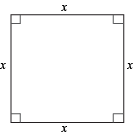Wow. What a square.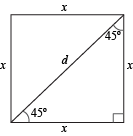Much better.

Now we have two 45-45-90 isosceles triangles, with diagonal d as our hypotenuse. A little known fact about these triangles: they're more magical than Gandalf from The Lord of the Rings. Don't believe us?See? Wizardly. They have special properties, too. If we throw the Pythagorean Theorem at them, we'll see why.

a2 + b2 = c2

Since c is d, we can substitute that in. The magical thing about these triangles, though, is that a and b equal each other. In our case, they're both x.

d 2 = x2 + x2
d 2 = 2x2

Taking the positive square root of both sides will tell us what the hypotenuse equals.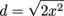Factoring whatever's under the square root will simplify things. Watch carefully. Things are about to get magical.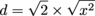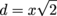One side to find them all:
One leg times root 2
Equals the hypotenuse
And that, we know, is true.

For every 45-45-90 triangle, one side can give us the lengths of all the sides. That's magical enough to make J.R.R. Tolkien proud.

### Sample Problem

What is the length of this triangle's legs?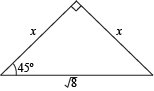Its hypotenuse is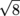, and like the magic poem says, we only need one side to figure out the others. As usual, we'll start with the formula we derived from the Pythagorean Theorem.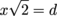Substitute in our hypotenuse for d.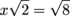Divide both sides by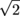.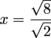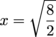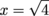x = 2

• ### 30-60-90 Triangles

The other magical right triangle comes from an equilateral triangle, or what might be better known as the Triforce from The Legend of Zelda.Not one of those is a right triangle, but if we cut them in half, they will be.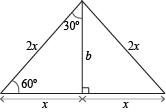Like the Gandalf triangle, knowing any side length of the Triforce triangle, which has angles of 30°, 60°, and 90°, can tell us the other sides. Magical, but just a smidgeon more work to do. Don't worry, it's nothing like trudging through Hyrule to rescue Princess Zelda.

We'll start as we did before, giving a shout out to Mr. Pythagoras.

a2 + b2 = c2

If we look at that hacked-up equilateral triangle, we can see that the hypotenuse of the right triangle equals twice the length of the shortest leg. After all, we did cut it exactly in half.

x2 + b2 = (2x)2

x2 + b2 = 4x2

Subtract x2 from both sides and then take the positive square root.

b2 = 3x2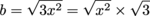Finally, we have our formula.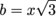Real magic does exist. The thing is, now we have three formulas to remember: one for each side of the triangle. That's the other reason we call it the Triforce triangle.

1. Short leg = x
2. Long leg =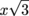3. Hypotenuse = 2x

Notice that the long leg of the triangle is not twice the length of the short leg. It's a really common mistake, but a quick look at our chopped-up Triforce will tell us why that's just not true. The hypotenuse is twice the length of the short leg.

Sometimes, knowing which side is the short leg and which is the long leg is confusing, but that's what angles are for. The long leg is opposite of the 60° angle, and the short leg is opposite of the 30° angle.

### Sample Problem

What are the sides of a 30-60-90 triangle that has a hypotenuse of 10?

This should be a piece of Hyrulean cake. If we know the length of the hypotenuse, we can figure out the rest. We'll start with the hypotenuse formula.

Hypotenuse = 2x
10 = 2x
x = 5

Since the value of x is the length of the short leg, we already have two out of three. All that's left is finding the long leg.

Long leg =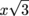Long leg =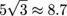Shazam. The sides are 5, 8.7, and 10.